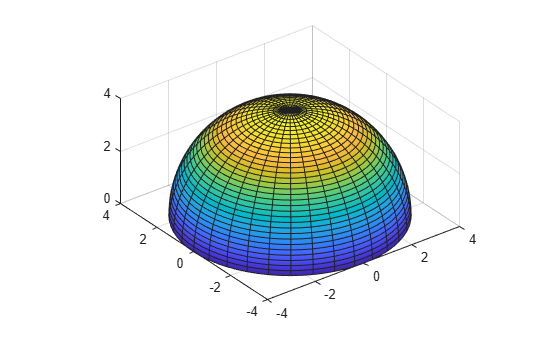Plotting in Spherical Coordinate System

This example shows how to plot a point in spherical coordinates and its projection to Cartesian coordinates.

In spherical coordinates, the location of a point $P$ can be characterized by three coordinates:

• the radial distance $\rho$

• the azimuthal angle $\theta$

• the polar angle $\varphi$The relationship between the Cartesian coordinates $\left(x,y,z\right)$ of the point $P$ and its spherical coordinates $\left(\rho ,\theta ,\varphi \right)$ are:

$\begin{array}{l}\mathit{x}=\rho \text{\hspace{0.17em}}\mathrm{sin}\text{\hspace{0.17em}}\varphi \text{\hspace{0.17em}}\mathrm{cos}\text{\hspace{0.17em}}\theta \text{\hspace{0.17em}}\\ \mathit{y}=\rho \text{\hspace{0.17em}}\mathrm{sin}\text{\hspace{0.17em}}\varphi \text{\hspace{0.17em}}\mathrm{sin}\text{\hspace{0.17em}}\theta \text{\hspace{0.17em}}\\ \mathit{z}=\rho \text{\hspace{0.17em}}\mathrm{cos}\text{\hspace{0.17em}}\varphi \end{array}$

Plot the point $P$ using plot3. You can adjust the location of the point by changing the values of rho, theta, and phi.

rho =0.8;
theta =1.2;
phi =0.75;
x = rho*sin(phi)*cos(theta);
y = rho*sin(phi)*sin(theta);
z = rho*cos(phi);
plot3(x,y,z,'ko','MarkerSize',10,'MarkerFaceColor','k')
hold on

Plot the line projection of the point $P$ onto the $z$-axis and the $xy$-plane using fplot3.

syms r s
xr = r*sin(phi)*cos(theta);
yr = r*sin(phi)*sin(theta);
zr = r*cos(phi);
fplot3(xr,yr,zr,[0 rho],'k')
fplot3(xr,yr,sym(0),[0 rho],'k')
fplot3(xr,yr,sym(z),[0 rho],'k--')
fplot3(sym(x),sym(y),rho*sin(s),[0 pi/2-phi],'k')

Plot the planes that show the span of the azimuthal angle $\theta$ and the polar angle $\varphi$.

syms s t
xa = rho*sin(s)*cos(t);
ya = rho*sin(s)*sin(t);
fsurf(xa,ya,0,[0 phi 0 theta],'FaceColor','b','EdgeColor','none')
syms u v
xp = u*sin(v)*cos(theta);
yp = u*sin(v)*sin(theta);
zp = u*cos(v);
fsurf(xp,yp,zp,[0 rho 0 phi],'FaceColor','g','EdgeColor','none')
xlabel('x')
ylabel('y')
zlabel('z')
view(115,30)
axis equal;
hold offSymbolic Math Toolbox Documentation

Mathematical Modeling with Symbolic Math Toolbox

Get examples and videos